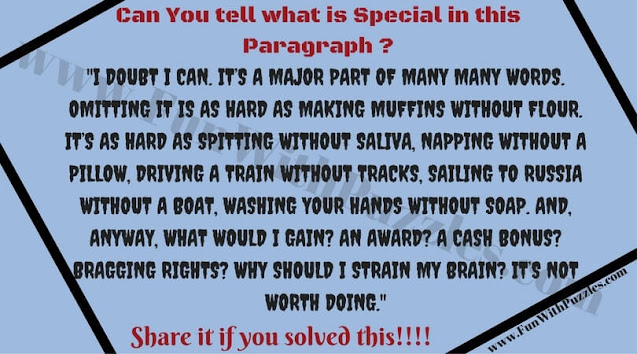We have Mathematical Puzzles in which one has to do lots of mathematical calculations to get the solution. Then we have Logical Puzzles in which one has to think logically to solve it. However, there are many Fun Puzzles which are neither Mathematical nor Logical. In these Puzzles, one has to think out of the box to solve these puzzles. These fun puzzles are definitely going to blow your mind. Let's see how many of these fun puzzles you can solve without looking at the answers?

Q1. Let's start with the first Fun Puzzle. Only 5% of Stanford University graduates figured it out! Can you answer all seven of the following questions with the same word?1. Can you solve this mind-blowing puzzle?

Think hard before looking at the answers :) !!!

OK, are you ready for the next question?
Here be go

2. Many of the times something unusual comes in front of our eyes but we cannot see anything special in this. Here is one such paragraph. Can you tell me What is special in this paragraph?2. Can you tell me What is special in this Paragraph?

Let's move to the next question which will make you think out of the box.3. Can you solve this out of box thinking puzzle?

Next question is a very interesting one. Again you will require out of the box thinking brain to solve this one.4. Can you solve this Out of Box Thinking Brain Teaser?

Last two puzzles are Word Fun Puzzles which will require knowledge of the English language to solve it. If you are not very much comfortable with the English language then you can skip these ones.5. Can you solve this Fun English Word Puzzle?6. Will you solve this Fun English Word Puzzle?

Do explore the more Fun Puzzles on this website as mentioned below
List of Fun Brain Teasers and Puzzles

1. Fun Brain Teasers For Kids, Teens and Adults with Answers to Challenge your Mind: Many of different types of Fun Brain Teasers which will keep challenging your mind.

2. English Word Puzzles for students with answers: It contains the English Word Puzzles to twist your mind.

3. Hidden Meaning Word Puzzles with Answers: These are the Rebus Puzzles in which your challenge is to guess the English word represented by the picture puzzle image.

4. Out of Box the Thinking Fun Brain Teasers to Challenge your Mind: These are the Fun Brain Teasers which will make you think out of the box.

-------------------------
Here is the answer to 9th Brain Teaser Question of post titled "Out of Box Thinking Fun Brain Teasers with answers to Challenge your Mind".

I have hidden this answer to avoid any spoiler. Do select the text below to read the answer.
---------------

If we number English alphabets to number in sequence like A=1, B=2, C=3 and so on then T=20.
Answer to this puzzle is the multiplication of these numbers
AT=1x20=20
BAT=2x1x20=40
CAT=3x1x20=60
---------------------

Unknown said...

Unknown said...

WHAT IF I HATE MY LIFE???

Unknown said...

you don't have to.

Unknown said...

no, you don't

Digital Education said...

if 1+2 =8 2+3=13 3+4=18 5+4=22 then 7+6=?

Rajesh Kumar said...

if 1+2 =8 2+3=13 3+4=18 5+4=22 then 7+6=32